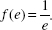# AP Calculus BC Question 277: Answer and Explanation

### Test Information

Question: 277

7. A relative maximum value of the function is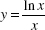is

• A. 1
• B. e
• C.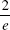• D.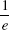(D) Here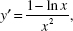which is zero for x = e. Since the sign of y changes from positive to negative as x increases through e, this critical value yields a relative maximum. Note that